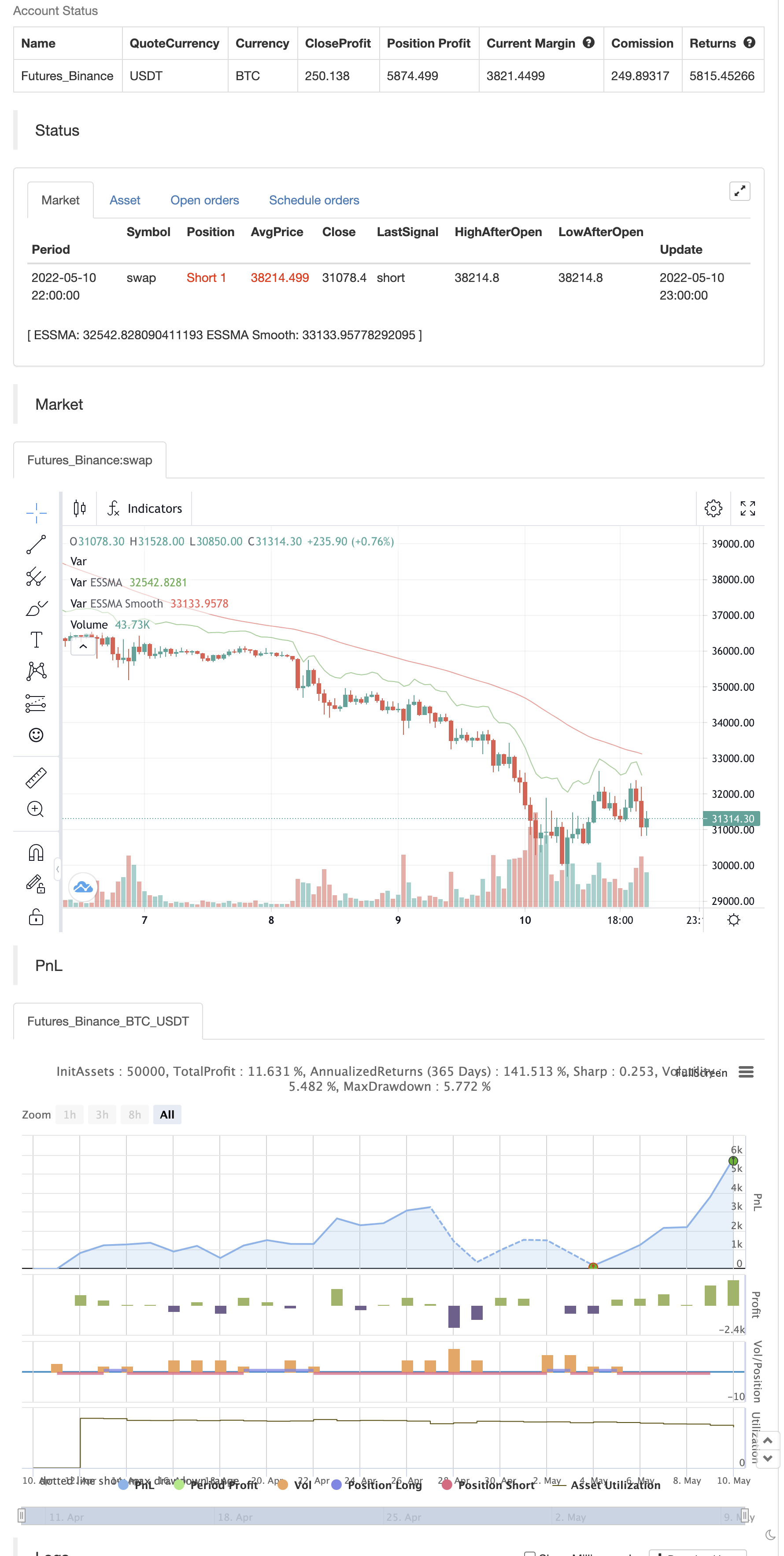# ESSMA

Author: ChaoZhang, Date: 2022-05-12 15:19:33
Tags: EMAsmaWMARMAATR

Concept:

There are a large number of moving averages available.

However, they are effective differently.

Trends confirmation and follow through requires a large number of moving averages to be used differently.

The concept here is to generate a combination moving average, each MA type can be weighted to provide a higher degree of confirmation of trend.

The weights are configurable in settings, and as a sample 50 length has been used.

ATR did not produce good result, so has been kept as optional.

The source can be modified.

The indicator provides a good Resistance support value in the larger time frame. And also provides a breakout and breakdown indication. Follow through is mostly effective.

The alert condition has been made such that it can directly be ported to discord.

For alerts one must configure their own message.```/*backtest
start: 2022-04-11 00:00:00
end: 2022-05-10 23:59:00
period: 1h
basePeriod: 15m
exchanges: [{"eid":"Futures_Binance","currency":"BTC_USDT"}]
*/

// This source code is subject to the terms of the Mozilla Public License 2.0 at https://mozilla.org/MPL/2.0/

//@version=5
indicator("ESSMA", overlay=true)
//inputs
source = input(close, "Source", group="Source")
length1 = input(50, "Length1", group = "Length")

w1 = input.float(2.0, "SMA Weight", group="Weights")
w2 = input.float(2.0, "EMA Weight", group="Weights")
w3 = input.float(2.0, "WMA Weight", group="Weights")
w4 = input.float(2.0, "SMMA Weight", group="Weights")
w5 = input.float(2.0, "RMA Weight", group="Weights")

useatr = input.bool(false, "Use ATR", group="ATR")

atrLen    = input.int(title="ATR Length", defval=14, group="ATR")

// functions

smma(src, length) =>
smma =  0.0
smma := na(smma) ? ta.sma(src, length) : (smma * (length - 1) + src) / length
smma

essma(src,length) =>
essma = 0.0

smma = smma(src * w4,length)
ema = ta.ema(src * w2, length)
sma = ta.sma(src * w1, length)
wma = ta.wma(src * w3, length)
rma = ta.rma(src * w5, length)

essma := (smma/w4+ema/w2+sma/w1 - wma/w3 - rma/w5 + open + close)/(3)
essma
// calucations

// atr and MAs

atr = ta.atr(atrLen)

usesource = useatr ? atr : source

essma1 = essma(usesource, length1)
sessma1 = ta.wma(essma1, length1)
// plots

p1 = plot(essma1, "ESSMA", color.green)

ps1 = plot(sessma1, "ESSMA Smooth", color.red)

bool up = na
bool down = na

if (ta.crossover(essma1,sessma1))
up := true

if (ta.crossunder(essma1, sessma1))
down := true

plotshape(up, style=shape.labelup, location = location.belowbar, color=color.lime, text="B", textcolor=color.black)
plotshape(down, style=shape.labeldown, location = location.abovebar, color=color.orange, text="S", textcolor=color.black)